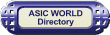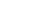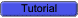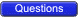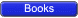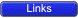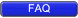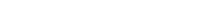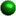Code Conversion

Converting from one code form to another code form is called code conversion, like converting from binary to decimal or converting from hexadecimal to decimal.Binary-To-Decimal Conversion

Any binary number can be converted to its decimal equivalent simply by summing together the weights of the various positions in the binary number which contain a 1.Binary Decimal 110112 24+23+01+21+20 =16+8+0+2+1 Result 2710andBinary Decimal 101101012 27+06+25+24+03+22+01+20 =128+0+32+16+0+4+0+1 Result 18110You should have noticed that the method is to find the weights (i.e., powers of 2) for each bit position that contains a 1, and then to add them up.Decimal-To-Binary ConversionThere are 2 methods:• Reverse of Binary-To-Decimal Method
• Repeat DivisionReverse of Binary-To-Decimal MethodDecimal Binary 4510 =32 + 0 + 8 + 4 +0 + 1 =25+0+23+22+0+20 Result =1011012Repeat Division-Convert decimal to binary

This method uses repeated division by 2.Convert 2510 to binaryDivision Remainder Binary 25/2 = 12+ remainder of 1 1 (Least Significant Bit) 12/2 = 6 + remainder of 0 0 6/2 = 3 + remainder of 0 0 3/2 = 1 + remainder of 1 1 1/2 = 0 + remainder of 1 1 (Most Significant Bit) Result 2510 = 110012The Flow chart for repeated-division method is as follows: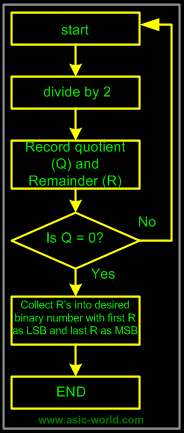Binary-To-Octal / Octal-To-Binary ConversionOctal Digit 0 1 2 3 4 5 6 7 Binary Equivalent 000 001 010 011 100 101 110 111Each Octal digit is represented by three binary digits.Example:

100 111 0102 = (100) (111) (010)2 = 4 7 28Repeat Division-Convert decimal to octalThis method uses repeated division by 8.Example: Convert 17710 to octal and binaryDivision Result Binary 177/8 = 22+ remainder of 1 1 (Least Significant Bit) 22/ 8 = 2 + remainder of 6 6 2 / 8 = 0 + remainder of 2 2 (Most Significant Bit) Result 17710 = 2618 Binary = 0101100012Hexadecimal to Decimal/Decimal to Hexadecimal ConversionExample:

2AF16 = 2 x (162) + 10 x (161) + 15 x (160) = 68710Repeat Division- Convert decimal to hexadecimal

This method uses repeated division by 16.Example: convert 37810 to hexadecimal and binary:Division Result Hexadecimal 378/16 = 23+ remainder of 10 A (Least Significant Bit)23 23/16 = 1 + remainder of 7 7 1/16 = 0 + remainder of 1 1 (Most Significant Bit) Result 37810 = 17A16 Binary = 0001 0111 10102Binary-To-Hexadecimal /Hexadecimal-To-Binary ConversionHexadecimal Digit 0 1 2 3 4 5 6 7 Binary Equivalent 0000 0001 0010 0011 0100 0101 0110 0111Hexadecimal Digit 8 9 A B C D E F Binary Equivalent 1000 1001 1010 1011 1100 1101 1110 1111Each Hexadecimal digit is represented by four bits of binary digit.Example:1011 0010 11112 = (1011) (0010) (1111)2 = B 2 F16Octal-To-Hexadecimal Hexadecimal-To-Octal Conversion• Convert Octal (Hexadecimal) to Binary first.
• Regroup the binary number by three bits per group starting from LSB if Octal is required.
• Regroup the binary number by four bits per group starting from LSB if Hexadecimal is required.Example:Convert 5A816 to Octal.Hexadecimal Binary/Octal 5A816 = 0101 1010 1000 (Binary) = 010 110 101 000 (Binary) Result = 2 6 5 0 (Octal)